#### You may also like### Consecutive Numbers

An investigation involving adding and subtracting sets of consecutive numbers. Lots to find out, lots to explore.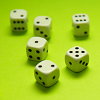### Roll These Dice

Roll two red dice and a green dice. Add the two numbers on the red dice and take away the number on the green. What are all the different possible answers?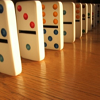### Domino Square

Use the 'double-3 down' dominoes to make a square so that each side has eight dots.

# Super Shapes

## Super Shapes

Each of the following shapes has a value: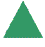$= 7$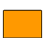$= 17$

The value of the red shapes changes in each of the following problems.
Can you discover its value in each problem, if the values of the shapes are being added together?

 (a)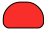$= 25$ (b)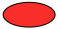$= 51$ (c)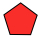$=136$ (d)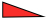$=48$ (e)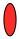$=100$

You could print off this sheet.pdf   of the problem.

### Why do this problem?

This problem provides an opportunity for pupils to practise using addition and subtraction, and it reinforces their inverse relationship. The problem gives learners chances to explain their working and it also helps them become familiar with the idea of a symbol (in this case a shape) representing a number.

### Possible approach

This problem would make a good starter. You could print off this sheet of the problem for children to work from and make sure that paper and/or mini-whiteboards are available for them to jot down any workings.

It would be important to talk about the methods that learners have used in each case and so invite a few children to share their way of working. The method is likely to differ depending on which problem is being solved and you can use this as an opportunity for the group to reflect on why particular approaches were chosen in each case. Emphasise that the method used is entirely up to the individual, but you may discuss which could be the most efficient.

### Key questions

How did you work this out?

### Possible extension

Shape Times Shape involves multiplication rather than addition and requires much more sophisticated reasoning.

### Possible support

You could provide calculators if you want children to focus on the method rather than the arithmetic.